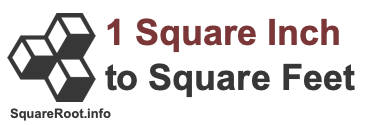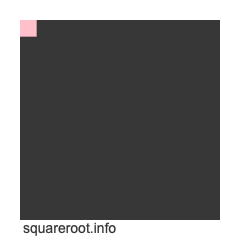1 square inch to square feetHere we will explain and show you how to convert 1 square inch to square feet. Before we continue, note that 1 square inch to square feet is the same as 1 sqin to sqft, 1 in2 to ft2, and 1 in² to ft².

To create a formula to calculate 1 square inch to square feet, we start with the fact that one inch equals one twelfth of a foot. Therefore, this formula is true:

Inches × (1/12) = Feet

However, we are dealing with square inches and square feet, which means inches and feet to the 2nd power. Thus, we take both sides of the formula above to the 2nd power to get this result:

Inches² × (1/12)² = Feet²
Inches² × (1/144) = Feet²
in² × (1/144) = ft²

Below is an illustration showing you how one square inch fits into one square foot. The cut out in pink in the top left corner is one square inch. You can fit 144 square inches into one square foot.Anyway, now we know that one square inch is equal to 1/144 square feet, and that the formula to convert square inches to square feet per the calculations above is as follows:

in² × (1/144) = ft²

When we enter 1 square inch into our formula, we get the answer to 1 square inch converted to square feet:

in² × (1/144) = ft²
1 × (1/144)
1 in² = 1/144 ft²
1 in² ≈ 0.006944 ft²

As you can see, shown above is the exact fractional answer to 1 square inch in feet and the rounded decimal answer to 1 square inch in feet.

Square Inches to Square Feet Converter
Please enter another square inches area in the box below to have it converted to square feet.

Convert  square inches to square feet.

1.01 square inches to square feet
Here is the next area in square inches on our list that we have converted to square feet.